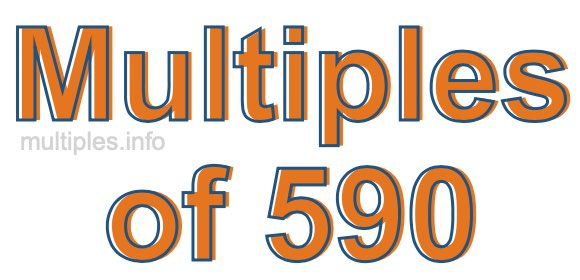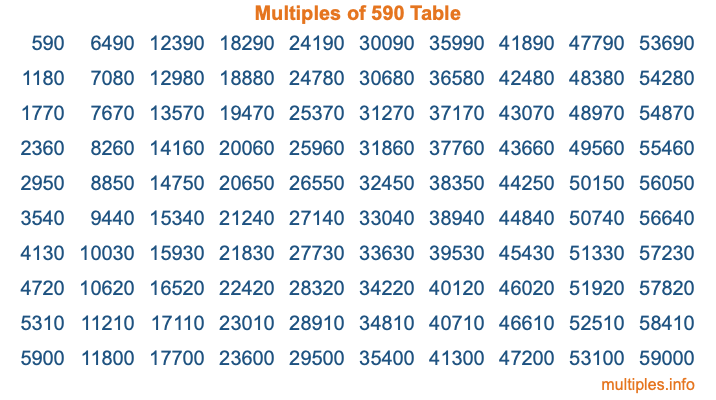Multiples of 590Welcome to the Multiples of 590 page. Here we will first teach you everything you will ever need to know about the multiples of 590, and then give you a study guide summary of everything we taught you to make sure you remember it all. Use this page to look up facts and learn information about the multiples of 590. This page will make you a multiples of five hundred ninety expert!

Definition of Multiples of 590
Multiples of 590 are all the numbers that when divided by 590 equal an integer. Each of the multiples of 590 are called a multiple. A multiple of 590 is created by multiplying 590 by an integer.

Therefore, to create a list of multiples of 590, you start with 1 multiplied by 590, then 2 multiplied by 590, then 3 multiplied by 590, and so on for as long as you want. Thus, the list of the first five multiples of 590 is 590, 1180, 1770, 2360, and 2950. To see a larger list of multiples of 590, see the printable image of Multiples of 590 further down on this page. We also have a category where you can choose any nth multiple of 590.

Multiples of 590 Checker
The Multiples of 590 Checker below checks to see if any number of your choice is a multiple of 590. In other words, it checks to see if there is any number (integer) that when multiplied by 590 will equal your number. To do that, we divide your number by 590. If the the quotient is an integer, then your number is a multiple of 590.

Is  a multiple of 590?

Least Common Multiple of 590 and ...
A Least Common Multiple (LCM) is the lowest multiple that two or more numbers have in common. This is also called the smallest common multiple or lowest common multiple and is useful to know when you are adding our subtracting fractions. Enter one or more numbers below (590 is already entered) to find the LCM.

Check out our LCM Calculator if you need more details about the Least Common Multiple or if you need the LCM for different numbers for adding and subtraction fractions.

nth Multiple of 590
As we stated above, 590 is the first multiple of 590, 1180 is the second multiple of 590, 1770 is the third multiple of 590, and so on. Enter a number below to find the nth multiple of 590.

th multiple of 590

Multiples of 590 vs Factors of 590
590 is a multiple of 590 and a factor of 590, but that is where the similarities end. All postive multiples of 590 are 590 or greater than 590. All positive factors of 590 are 590 or less than 590.

Below is the beginning list of multiples of 590 and the factors of 590 so you can compare:

Multiples of 590: 590, 1180, 1770, 2360, 2950, etc.

Factors of 590: 1, 2, 5, 10, 59, 118, 295, 590

As you can see, the multiples of 590 are all the numbers that you can divide by 590 to get a whole number. The factors of 590, on the other hand, are all the whole numbers that you can multiply by another whole number to get 590.

It's also interesting to note that if a number (x) is a factor of 590, then 590 will also be a multiple of that number (x).

Multiples of 590 vs Divisors of 590
The divisors of 590 are all the integers that 590 can be divided by evenly. Below is a list of the divisors of 590.

Divisors of 590: 1, 2, 5, 10, 59, 118, 295, 590

The interesting thing to note here is that if you take any multiple of 590 and divide it by a divisor of 590, you will see that the quotient is an integer.

Multiples of 590 Table
Below is an image of the first 100 multiples of 590 in a table. The table is in chronological order, column by column. The first column has the first ten multiples of 590, the second column has the next ten multiples of 590, and so on.The Multiples of 590 Table is also referred to as the 590 Times Table or Times Table of 590. You are welcome to print out our table for your studies.

Negative Multiples of 590
Although not often discussed or needed in math, it is worth mentioning that you can make a list of negative multiples of 590 by multiplying 590 by -1, then by -2, then by -3, and so on, to get the following list of negative multiples of 590:

-590, -1180, -1770, -2360, -2950, etc.

Multiples of 590 Summary
Below is a summary of important Multiples of 590 facts that we have discussed on this page. To retain the knowledge on this page, we recommend that you read through the summary and explain to yourself or a study partner why they hold true.

There are an infinite number of multiples of 590.

A multiple of 590 divided by 590 will equal a whole number.

590 divided by a factor of 590 equals a divisor of 590.

The nth multiple of 590 is n times 590.

The largest factor of 590 is equal to the first positive multiple of 590.

590 is a multiple of every factor of 590.

590 is a multiple of 590.

A multiple of 590 divided by a divisor of 590 equals an integer.

590 divided by a divisor of 590 equals a factor of 590.

Any integer times 590 will equal a multiple of 590.

Multiples of a Number
Here you can get the multiples of another number, all with the same attention to detail as we did for multiples of 590 on this page.

Multiples of
Multiples of 591
Did you find our page about multiples of five hundred ninety educational? Do you want more knowledge? Check out the multiples of the next number on our list!